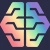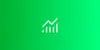PromptBaseExplore
ModelsMidjourneyDALL·EStable DiffusionGPTArt & IllustrationsAnimeCartoonPaintingIllustrationUnique StylesLogos & IconsLogoIconGraphics & DesignPatternProduct DesignProfile PictureProductivity & WritingProductivityWritingCodingMarketing & BusinessMarketingBusinessSocial MediaPhotographyPhotographyPhotography StyleGames & 3D3DFun & GamesVideo Game ArtGeneral
Home
Marketplace
Generate
Hire
Sell
🤖 GPT# Data Analysis Tool5Favorites
97Views
TestedTips🔓📊🔍 Unlock the power of data analysis with this prompt! 🚀 Perfect for beginners, it offers a deep dive into the field, covering essential skills like statistical analysis and data modeling. 💻 Plus, it has two functions - it can either analyze your data for you or teach you how to do it effectively. 🤓 Get started today and make informed decisions like a pro! 💪
...more
\$4.99
6 months ago

#### Prompt Details

Model
Chat - GPT-3.5 (gpt-3.5-turbo)
Token size
409 (\$0.0008 / call)
Example input
Example 1: Data Analysis Example 2: Explanation on How To Analyze Data
Example output
--Data Analysis-- Thank you for providing the data. Based on the data provided, we can analyze the number of steps taken over a 50-day period. Here are some observations we can make: The average number of steps taken per day over the 50-day period is 6,650. The maximum number of steps taken in a single day is 9,876. The minimum number of steps taken in a single day is 1,893. There appears to be some variation in the number of steps taken from day to day, with some days having significantly higher or lower numbers of steps than others. There are several days where the number of steps taken is higher than the average, including days 2, 6, 7, 9, 11, 12, 13, 16, 17, 19, 22, 23, 27, 28, 29, 31, 32, 33, 36, 37, 39, 42, 43, 46, and 47. Similarly, there are several days where the number of steps taken is lower than the average, including days 1, 3, 4, 5, 8, 10, 14, 15, 18, 20, 21, 24, 25, 26, 30, 34, 35, 38, 40, 41, 44, 45, 48, and 50. Based on this analysis, we can conclude that there is some variation in the number of steps taken from day to day, and that some days are more active than others. To further analyze the data, we could plot the number of steps taken over time to see if there are any trends or patterns in the data. --Explanation on How To Analyze Data-- To analyze the data, you will need to perform some basic statistical analysis. Here are some steps you can take: Calculate the mean (average) number of steps per day: Add up the total number of steps taken over the month and divide by the number of days. In this case, we would add up all the steps (365,684) and divide by 50 (the number of days), which gives us an average of 7313.68 steps per day. Calculate the median number of steps per day: Sort the daily step counts in ascending order and find the middle number. If there are an even number of data points, take the average of the two middle numbers. In this case, the median is 7654. Calculate the range of step counts: Find the difference between the highest and lowest values. In this case, the range is 7978 (9321 - 1343). Calculate the standard deviation of the step counts: This measures how spread out the data is from the mean. A higher standard deviation indicates more variation in the data. You can use a spreadsheet software or a programming language like Python to calculate the standard deviation. In this case, the standard deviation is 2478.67. Visualize the data: Creating a histogram or a line chart is a great way to visually analyze the data. A histogram will show you the frequency distribution of the step counts, while a line chart will show you the trend over time. By analyzing the data, you can get a better understanding of the overall pattern and trends in the step counts over the 50-day period. You can also use this information to set goals for increasing your step count or to identify patterns in your physical activity that you can adjust to improve your health.